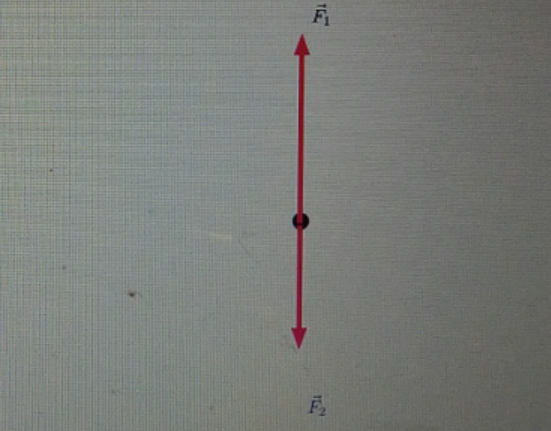# Problem: Add a third force that will cause the object to remain at rest. Label the new force  F⃗3.Draw the vector starting at the black dot. The location, orientation, and length of the vector will be graded. You can move the vectors  F⃗1 and  F⃗2 to construct the required vector, but be sure to return them into their initial positions before submitting the answer.

###### FREE Expert Solution

Newton's second law:
$\overline{){\mathbf{F}}{\mathbf{=}}{\mathbf{ma}}}$

F2 is less than F1.

At rest,

ΣF = F1 - F2 - F3 = 0

84% (205 ratings)###### Problem Details

Add a third force that will cause the object to remain at rest. Label the new force  F3.

Draw the vector starting at the black dot. The location, orientation, and length of the vector will be graded. You can move the vectors  F1 and  F2 to construct the required vector, but be sure to return them into their initial positions before submitting the answer.